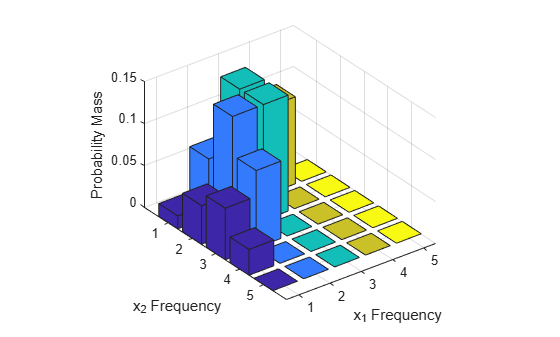## Multinomial Probability Distribution Functions

This example shows how to generate random numbers and compute and plot the pdf of a multinomial distribution using probability distribution functions.

### Step 1. Define the distribution parameters.

Create a vector `p` containing the probability of each outcome. Outcome 1 has a probability of 1/2, outcome 2 has a probability of 1/3, and outcome 3 has a probability of 1/6. The number of trials in each experiment `n` is 5, and the number of repetitions of the experiment `reps` is 8.

```p = [1/2 1/3 1/6]; n = 5; reps = 8;```

### Step 2. Generate one random number.

Generate one random number from the multinomial distribution, which is the outcome of a single trial.

```rng('default') % For reproducibility r = mnrnd(1,p,1)```
```r = 1×3 0 1 0 ```

The returned vector `r` contains three elements, which show the counts for each possible outcome. This single trial resulted in outcome 2.

### Step 3. Generate a matrix of random numbers.

You can also generate a matrix of random numbers from the multinomial distribution, which reports the results of multiple experiments that each contain multiple trials. Generate a matrix that contains the outcomes of an experiment with `n = 5` trials and `reps = 8` repetitions.

`r = mnrnd(n,p,reps)`
```r = 8×3 1 1 3 3 2 0 1 1 3 0 4 1 5 0 0 1 2 2 3 1 1 3 1 1 ```

Each row in the resulting matrix contains counts for each of the $k$ multinomial bins. For example, in the first experiment (corresponding to the first row), one of the five trials resulted in outcome 1, one of the five trials resulted in outcome 2, and three of the five trials resulted in outcome 3.

### Step 4. Compute the pdf.

Since multinomial functions work with bin counts, create a multidimensional array of all possible outcome combinations, and compute the pdf using `mnpdf`.

```count1 = 1:n; count2 = 1:n; [x1,x2] = meshgrid(count1,count2); x3 = n-(x1+x2); y = mnpdf([x1(:),x2(:),x3(:)],repmat(p,(n)^2,1));```

### Step 5. Plot the pdf.

Create a 3-D bar graph to visualize the pdf for each combination of outcome frequencies.

```y = reshape(y,n,n); bar3(y) set(gca,'XTickLabel',1:n); set(gca,'YTickLabel',1:n); xlabel('x_1 Frequency') ylabel('x_2 Frequency') zlabel('Probability Mass')```The plot shows the probability mass for each possible combination of outcomes. It does not show ${x}_{3}$ , which is determined by the constraint ${x}_{1}+{x}_{2}+{x}_{3}=n$ .